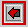# Regular Satellite Calculator### Satellite Variables

 pi ~ 3.141592654 G = gravitational constant c = speed of light Msun = Mass of Sun Rsun = Radius of Sun Lsun = Luminosity of Sun m2AU = meters to AU AU2m = AU to meters s2yr = seconds to years s2hr = seconds to hours r2d = radians to degrees d2r = degrees to radians

 Mp = mass ap = semimajor axis Rp = radius rhop = density Vop = orbital velocity ep = eccentricity J2 = oblateness tp = tilt

 M = mass R = radius a = Semimajor axis rho = density e = eccentricity i = inclination Vo = Orbital Velocity Hr = Hill Radius Ve = Escape Velocity Po = Orbital Period gs = Surface gravity Rsyn = Synchronous Orbit

Try the Planetary Calculator and the Irregular Satellite Calculator!Return to the Astronomy Workshop

Regular Satellite Calculator written by Dr. Douglas P. Hamilton and Amanda L. Proctor